# Python Programming Fundamentals — Loan Payment ScheduleSometimes, when learning a new language it is helpful to break down a problem that you are familiar with, and apply the concepts of the programming language to that problem. An example of this is the temperature converter we did a few articles back.

In this article we are going to do the same thing, except this time we are going to build a Loan Payment Schedule (also known as an Amortization Schedule).

Quick note: I'm not a financial advisor, there are many factors that go into loan payment calculation that are not considered here. This exercise should only be used to approximate payment amounts and timelines.

We are going to be pulling together many items we've touched on before including functions, loops, conditional statements, and importing libraries. Before we begin, there is one library that you may not have already installed, but it will make viewing the results of our program much nicer. We are going to use the python package manager pip to install a library called BeautifulTable. In order to do this, open a command prompt and type

``````pip3 install beautifultable
``````

pip3 is the package manager for Python3, and BeautifulTable is only supported on Python3.4 and up.

Once you have that installed you are ready to go.

### Payment Calculation

If you have ever had a loan of any type, you have made payments on it at one time or another. There is a formula for calculating this payment which is: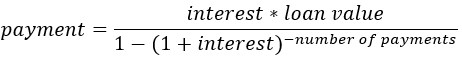Loan Payment Formula

Interest is the rate you pay towards the loan, here it will be used as a decimal (so for example: 5% is .05). The number of payments will be determined by how many years the loan is for multiplied by 12 (one payment per month). If your loan is for less than a year, you can use a fraction (i.e. 9 months is .75).

With this in hand, we can start building our program!

We'll create a function called amortize and give it a few arguments to cover the necessary variables for our formula. We'll also create some variables to convert our user input into something that can be directly used in the formula:

``````def amortize(value,rate,pmt_yrs):
intrate = rate/12.0
totalpmts = pmt_yrs *12
payment = (intrate * value) / ( 1 - pow(1+intrate,-totalpmts))
``````

As it stands, you can call this function with the required arguments and print out payment. This is pretty helpful, but we want a full payment schedule so we still have some work to do.

Next we're going to use a while loop to calculate each month's payment:

``````while value > 0:
interest = (value * intrate)
principle = payment - interest

if value - payment < 0:
principle = value

value = value - principle
``````

Interest can be calculated by multiplying the interest rate and the current loan value. Each month this number will be different, so we include it in the loop. Principle is the actual amount of the payment that goes towards the loan balance and is found by subtracting the calculated interest from the payment.

Our loop condition states that we are going to loop as long as the value of the loan is greater than \$0. The easiest check here is to see whether the next payment will cause the loan to be less than 0, and if so, then the principle payment of this month will be equal to the loan value.

Finally, the loan value is updated by subtracting the current months principle payment.

Here's what we have so far:

``````def amortize(value,rate,pmt_yrs):
intrate = rate/12.0
totalpmts = pmt_yrs *12
payment = (intrate * value) / ( 1 - pow(1+intrate,-totalpmts))

while value > 0:
interest = (value * intrate)
principle = payment - interest

if value - payment < 0:
principle = value

value = value - principle
``````

This will (silently) compute the loan payment schedule over the lifetime of the loan. For this to be useful, we are going to need some output. This is where BeautifulTable comes in.

### Building the Table

At the top of your script, use the following to include the BeautifulTable library functions in your code:

``````from beautifultable import BeautifulTable
``````

Now we can create an instance of the BeautifulTable class. A class is an object oriented programming template for implementing different objects in programming code. Here we are going to create a BeautifulTable object by calling the class's constructor. A constructor ensures that all necessary variables and data members are available to the new object.

``````table = BeautifulTable()
``````

That's it! Now we have a fully created table object of the BeautifulTable class that we can use.

If you'd like to check out all that BeautifulTable can do, check out its PyPi page
https://pypi.org/project/beautifultable/

Our table needs a header across all of the columns so we create one like this:

``````table.columns.header=["MONTH","LOAN VALUE","PAYMENT","INTEREST","PRINCIPLE","NEW VALUE"]
``````

Looks a lot like a list right? Here we use the class data members “columns” and “header” to tell table how we want our data set up.

We're going to need to know which month we're on, so we create a counter variable and initialize it to 0 (so the first payment, will be in Month 1).

Finally, the data needs to be put into the table, so we'll use the “rows” data member and call it's “append” method to insert our data into that row of the table.

``````table.rows.append([n,value,payment,interest,principle,value-principle)])
``````

Now let's take a look at our code: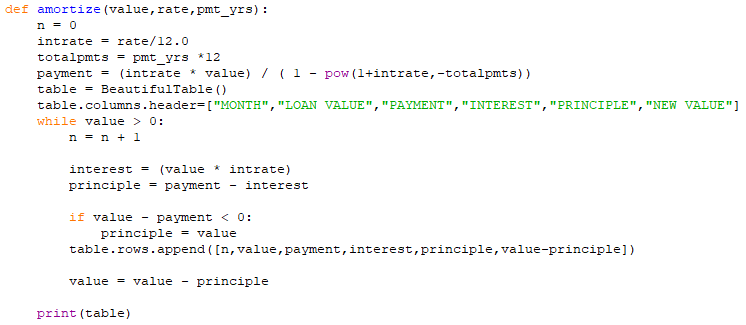Current Amortization Function

And if we run it with some data, we can see that it works fairly well (only a portion of the output is shown):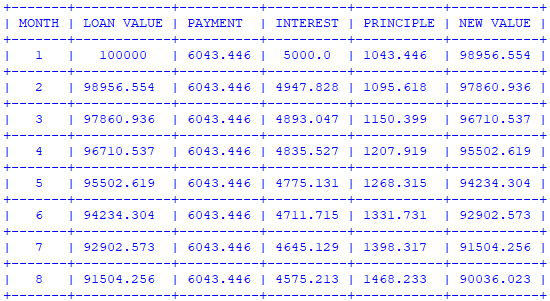Partial Amortization Schedule

You could stop here, and have a fully functional loan amortization schedule program. However, there are a few quality of life upgrades we can make to enhance the usability of this program.

One downside to the current payment schedule is we don't know what currency that is. 100,000 could be a lot, or not very much depending on the location of the user. Luckily python includes a library to help with localization.

The locale library contains many functions to help manage data from different regions of the world. For our purposes we are going to take advantage of the locale.currency method within that library to format our currency to something that looks more familiar. Mine will show up as USD (\$), yours may vary depending on your environment variables.

``````import locale
locale.setlocale( locale.LC_ALL, '' )
``````

The call to setlocale sets the default locale based on your LANG environment variable.

Now we can modify our amortize function with locale.currency: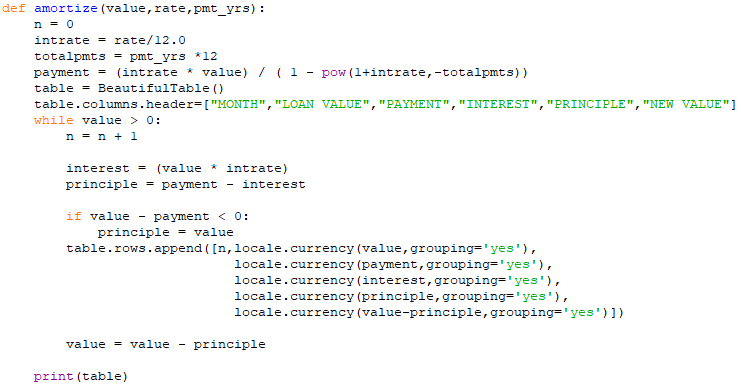Updated amortize function

We simply placed the function call within the row append for each monetary item, and converted it to a location based currency with digit grouping. Here's the new print out.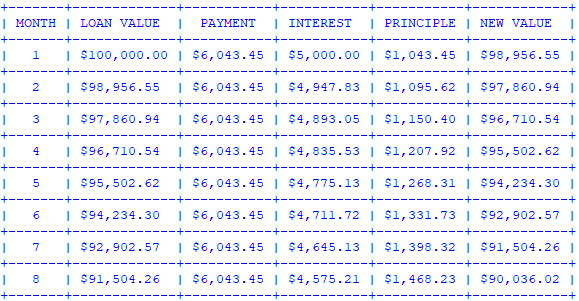Location based currency formatting with grouping

### Command Line Arguments

As if that wasn't enough, we can extend this a tad bit further. We can make use of the `_argparse_` library in order to reuse this application without needing to make changes to the code for different loan amounts, interest rates, or payment lengths.

There are many different ways to handle command line arguments in python, and `argparse` is only one such way. To get started we import the library like usual:

``````import argparse
``````

Next we need to create an ArgumentParser object. To do that we will call it's constructor much like we saw earlier with BeautifulTable. Then we will add a few arguments to our program. One for each variable of our function.

``````parser = argparse.ArgumentParser()
parser.add_argument("rate",help="Interest as a decimal(5% = .05)
``````

Failure to provide all of these items on the command line will result in a message similar to the following:

`_amortize.py: error: the following arguments are required: value, rate, years_`

Now we need to assign these command line arguments to variables that we can pass to our function. We do this by first parsing the arguments provided:

``````args = parser.parse_args()
``````

Now we can access the data elements of our args object:

``````value = args.value
rate = args.rate
pmt_yrs = args.years
``````

and finally call our `amortize` function with those variables:

``````amortize(value, rate, pmt_yrs)
``````

By wrapping all of this into a main() function, we have a nice starting spot for our program. Note that I also cast each of the inputs as a float data type. This is to aid the calculation of fractional years, as well as the division involved with computing interest rates.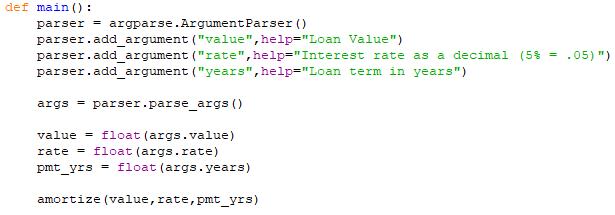main() function

If you are coding along in IDLE then you'll notice if you run the program with F5 you will get the error mentioned above. This is because no command line arguments were passed to the program, but it will still work. On the prompt you can simply call the function as your code would. Of course, if you're running this from a command prompt you should have no issues.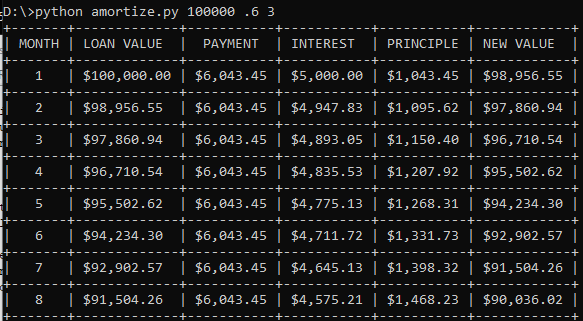Putting everything together, we get the full loan payment schedule program below: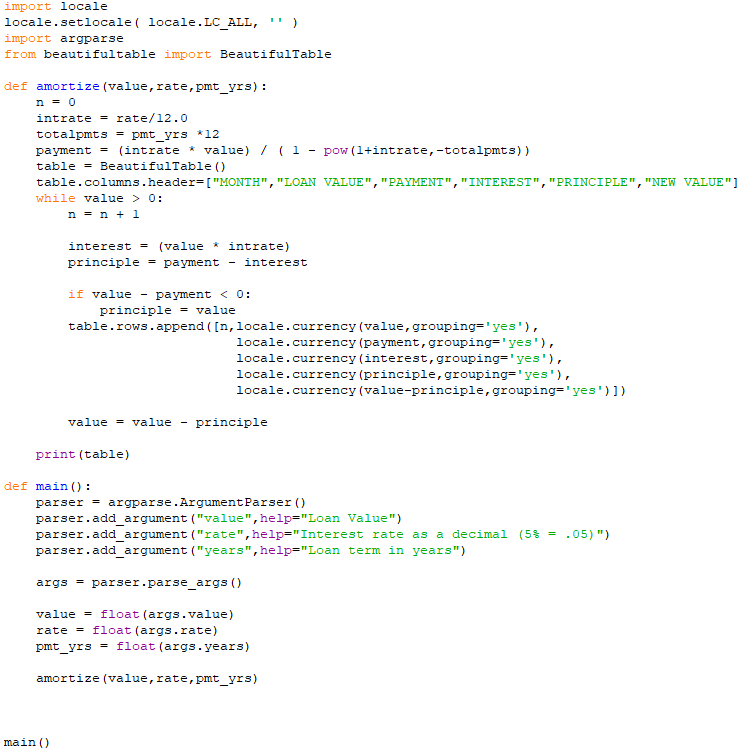Full Program

Hopefully you enjoyed working through this example program, and will be able to apply the concepts here to other problems. If you enjoyed this content, please consider supporting over at Patreon or BuyMeACoffee.